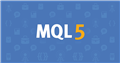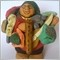• Get access

# Moving Horizontal line in the chart275

```   preblueline=data[pos];
if(data[pos]>Open)
{
blueline=data[pos];
}
if(data[pos]<Open)
{
blueline=data[pos];
}
if (preblueline!=blueline){preblueline=blueline;}```

Hi Friends,

I draw a horizontal line the chart say at price 1.14500 ( Previous Value)

Now the market moves the my new horizontal line is 1.12345 (Current Value)

I want to show these in the Chart like

Previous Position of Pivot Line is x.xxxxx Present Position is x.xxxxx

I am trying lot of codes but it shows current pivot value for both. Though i am pro coder.Documentation on MQL5: Constants, Enumerations and Structures / Objects Constants / Object Types
• www.mql5.com
When a graphical object is created using the ObjectCreate() function, it's necessary to specify the type of object being created, which can be one of the values of the ENUM_OBJECT enumeration. Further specifications of object properties are possible using functions for working with graphical objects.2939

Vijay Akash T P:

Hi Friends,

I draw a horizontal line the chart say at price 1.14500 ( Previous Value)

Now the market moves the my new horizontal line is 1.12345 (Current Value)

I want to show these in the Chart like

Previous Position of Pivot Line is x.xxxxx Present Position is x.xxxxx

I am trying lot of codes but it shows current pivot value for both. Though i am pro coder.

https://www.mql5.com/en/docs/constants/objectconstants/enum_object/obj_hlineDocumentation on MQL5: Constants, Enumerations and Structures / Objects Constants / Object Types / OBJ_HLINE
• www.mql5.com
//| Create the horizontal line                                       |                 price=0,                         width=1,            //| Move horizontal line                                             | //| Delete a horizontal line                                         |...275

Fernando Morales:

https://www.mql5.com/en/docs/constants/objectconstants/enum_object/obj_hline

This is not what i am looking boss. I created a line at a price (X) for given condition. Later stage the price (X) changes for the given condition.

Here there are two value for X.

Now the problem is I want to recall

Previous value is X

Present value is XModerator
21036

```   preblueline=data[pos];
if(data[pos]>Open)
{
blueline=data[pos];
}
if(data[pos]<Open)
{
blueline=data[pos];
}
if (preblueline!=blueline){preblueline=blueline;}```

Please explain what you are trying to achieve with this block of code.275

Keith Watford:

Please explain what you are trying to achieve with this block of code.

I created a line at a price (X) for given condition. Later stage the price (X) changes for the given condition.

Here there are two value for X. which i would call as Previous value and present value.

Now the problem is I want to store them separaetely and recall later

Previous value is X

Present value is X20410

You need code that saves the previous value when you update the current value?275

William Roeder:
You need code that saves the previous value when you update the current value?
``` preblueline=data[pos];
if(data[pos]>Open)
{
blueline=data[pos];
}
if(data[pos]<Open)
{
blueline=data[pos];
}
if (preblueline!=blueline){preblueline=blueline;}```

this is not working broModerator
21036

Vijay Akash T P:

I created a line at a price (X) for given condition. Later stage the price (X) changes for the given condition.

Here there are two value for X. which i would call as Previous value and present value.

Now the problem is I want to store them separaetely and recall later

Previous value is X

Present value is X

I am not sure what you are trying to do.

```   preblueline=data[pos];
if(data[pos]>Open)
{
blueline=data[pos];
}
if(data[pos]<Open)
{
blueline=data[pos];
}
if (preblueline!=blueline){preblueline=blueline;}```

Can be simplified to

```   preblueline=data[pos];
if(data[pos]!=Open)
blueline=data[pos];
else
preblueline=blueline;```275

Keith Watford:

I am not sure what you are trying to do.

Can be simplified to

i am getting the same value for both the lines. What i want is Preblueline is previous value and Blue is current value. Help me pleaseModerator
21036

Vijay Akash T P:

i am getting the same value for both the lines. What i want is Preblueline is previous value and Blue is current value. Help me please

The problem is that you are talking about price(x) and you don't use x in your code.

What is data[pos] ?275

Keith Watford:

The problem is that you are talking about price(x) and you don't use x in your code.

What is data[pos] ?

To make it clear below actual issue:

close price on a given condition is 1.10876 - previous value
After sometime close price on a given condition is 1.11768 - present value
I want store these values and recall it.

After this present value will change, then present has to be previous value and newly changed value is present value

Cycle goes on. Hope I tried to